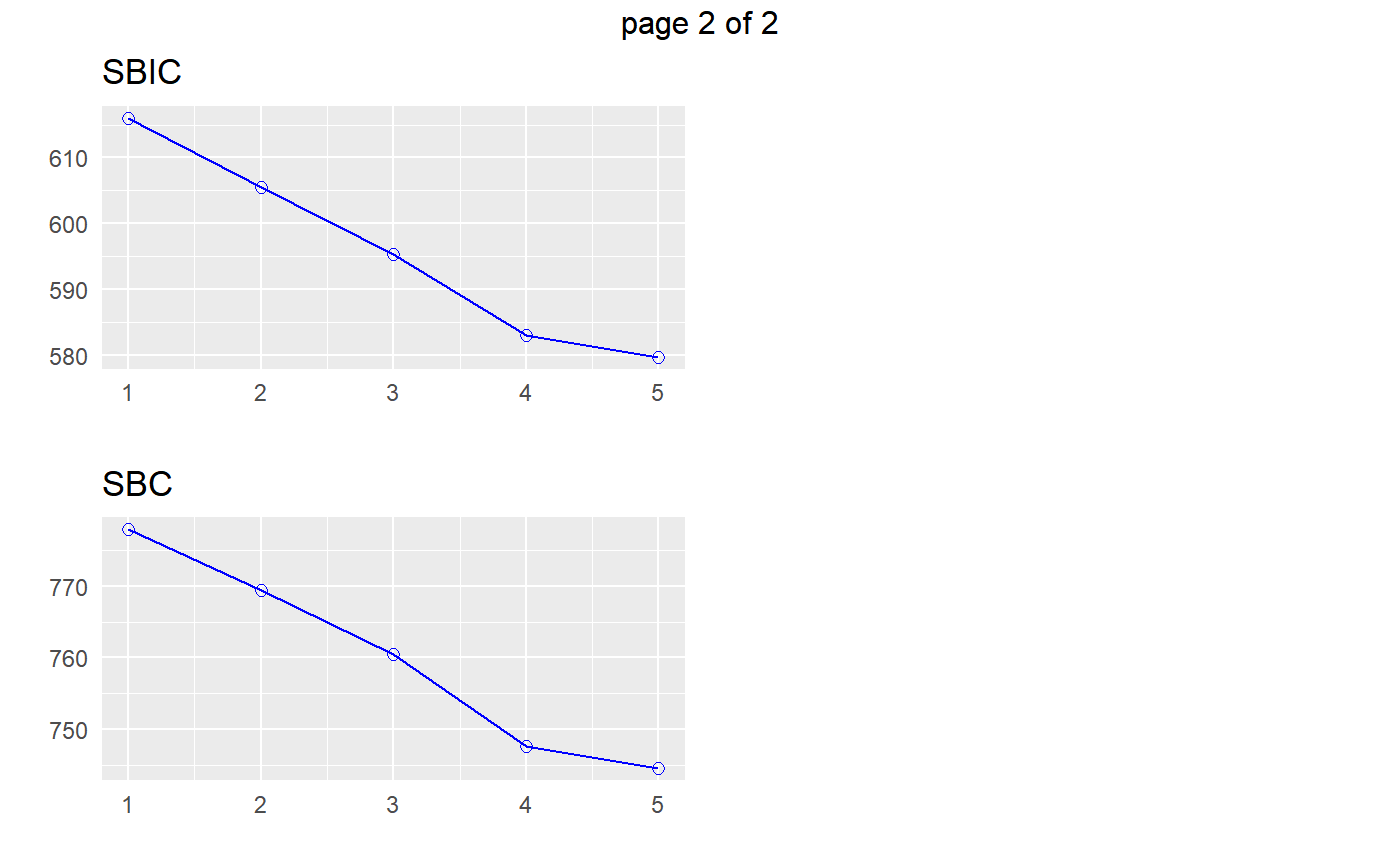Build regression model from a set of candidate predictor variables by entering and removing predictors based on p values, in a stepwise manner until there is no variable left to enter or remove any more.

ols_step_both_p(model, ...)

# S3 method for default
ols_step_both_p(
model,
pent = 0.1,
prem = 0.3,
progress = FALSE,
details = FALSE,
...
)

# S3 method for ols_step_both_p
plot(x, model = NA, print_plot = TRUE, ...)

## Arguments

model An object of class lm; the model should include all candidate predictor variables. Other arguments. p value; variables with p value less than pent will enter into the model. p value; variables with p more than prem will be removed from the model. Logical; if TRUE, will display variable selection progress. Logical; if TRUE, will print the regression result at each step. An object of class ols_step_both_p. logical; if TRUE, prints the plot else returns a plot object.

## Value

ols_step_both_p returns an object of class "ols_step_both_p". An object of class "ols_step_both_p" is a list containing the following components:

model

final model; an object of class lm

orders

candidate predictor variables according to the order by which they were added or removed from the model

method

addition/deletion

steps

total number of steps

predictors

variables retained in the model (after addition)

rsquare

coefficient of determination

aic

akaike information criteria

sbc

bayesian information criteria

sbic

sawa's bayesian information criteria

adjr

adjusted r-square

rmse

root mean square error

mallows_cp

mallow's Cp

indvar

predictors

## Deprecated Function

ols_stepwise() has been deprecated. Instead use ols_step_both_p().

Chatterjee, Samprit and Hadi, Ali. Regression Analysis by Example. 5th ed. N.p.: John Wiley & Sons, 2012. Print.

## Examples

# stepwise regression
model <- lm(y ~ ., data = surgical)
ols_step_both_p(model)#>
#>                                 Stepwise Selection Summary
#> ------------------------------------------------------------------------------------------
#>                         Added/                   Adj.
#> Step     Variable      Removed     R-Square    R-Square     C(p)        AIC         RMSE
#> ------------------------------------------------------------------------------------------
#>    1    liver_test     addition       0.455       0.444    62.5120    771.8753    296.2992
#>    2     alc_heavy     addition       0.567       0.550    41.3680    761.4394    266.6484
#>    3    enzyme_test    addition       0.659       0.639    24.3380    750.5089    238.9145
#>    4      pindex       addition       0.750       0.730     7.5370    735.7146    206.5835
#>    5        bcs        addition       0.781       0.758     3.1920    730.6204    195.4544
#> ------------------------------------------------------------------------------------------
# stepwise regression plot
model <- lm(y ~ ., data = surgical)
k <- ols_step_both_p(model)
plot(k)
# final model
k\$model#>
#> Call:
#> lm(formula = paste(response, "~", paste(preds, collapse = " + ")),
#>     data = l)
#>
#> Coefficients:
#> (Intercept)   liver_test    alc_heavy  enzyme_test       pindex          bcs
#>   -1178.330       58.064      317.848        9.748        8.924       59.864
#>